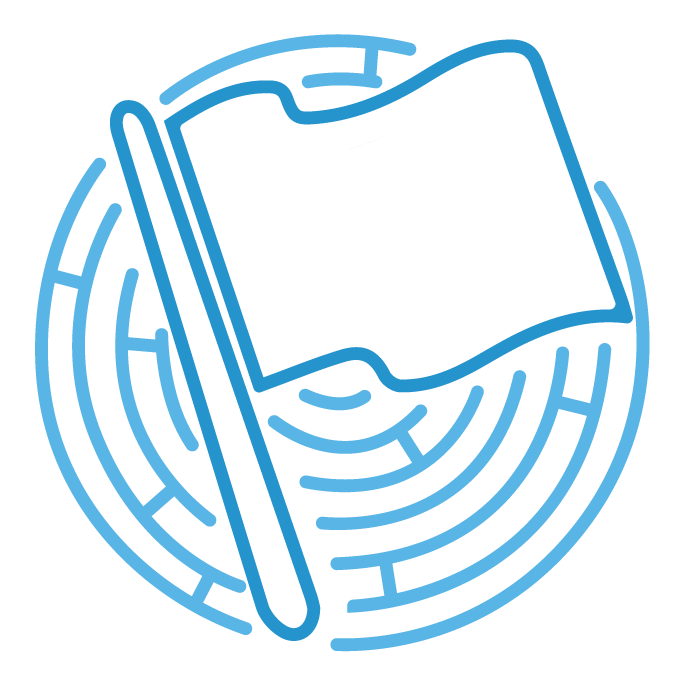/ #Harekaze

# A crypto-reversing challenge

Summary: Gocha was a crypto-reversing challenge for 100 points. We were given the following code in python 3. This code is for an online lottery system which asks provides the user with 3 choices of seemingly random numbers, one of which is the winner key and the other two is losing keys. So the probability of winning is 33.3%. However, the user has to win 90% of times for playing at least 30 times. So the probability of winning is a little bit higher than `3.6e-15`.

``````

# Python Version: 3.5.2
# -*- coding: utf-8 -*-
from Crypto.Util.number import *
from gmpy2 import *
from flag import FLAG
from signal import *

def main():
signal(SIGALRM, lambda: None)
alarm(60)
print('[*] 🎉 WELCOME TO HAREKAZE-CTF LOTTERY CHALLENGE 🎉')
print('[*] to ensure fairness, we use a public-key 🔑 cryptosystem 🔒')
win = 0

for round_ in range(1, 10000):
print()
print('[*] ROUND %d' % round_)

# generate params
p = [ getPrime(1024) for _ in range(3) ]
q = [ getPrime(1024) for _ in range(3) ]
n = [ p[i] * q[i] for i in range(3) ]
e = 65537
d = [ invert(e, (p[i] - 1) * (q[i] - 1)) for i in range(3) ]
m = [ bytes_to_long(['WIN 💎', 'LOSE 💩'][bool(i)].encode()) for i in range(3) ]
c = [ pow(m[i], e, n[i]) for i in range(3) ]
f = sorted(range(3), key=lambda x: c[x])

# receive the choice
for x, i in enumerate(f):
print('    🔒 %d. (%#x, %d, %#x)' % (x + 1, n[i], e, c[i]))
print('[*] select:')
k = f[int(input('>>> ')) - 1]

# check the result
result = long_to_bytes(pow(c[k], d[k], n[k])).decode()
print('[*] result:', result)
if 'WIN 💎' in result:
print('[+] you win 🎉')
win += 1
else:
print('[!] you lose')

# send the witnesses
print('[*] here are the keys. please ensure that there is no cheating')
for i in range(3):
print('    🔑 %d. %d' % (i + 1, d[i]))

if round_ >= 30 and win / round_ >= 0.9:
print('[+] you got the flag 🏁:', FLAG)
break

if __name__ == '__main__':
main()

``````

Checking the code we notice that the "winning" string has the gem unicode embedded in it and the win/lose is decoded based on:

``````result = long_to_bytes(pow(c[k], d[k], n[k])).decode()
``````

Based on the following line we are provided with the c[i] and n[i] but not with the d[i], which seems to be required for decoding the winner key:

``````print('    🔒 %d. (%#x, %d, %#x)' % (x + 1, n[i], e, c[i]))
``````

However, instead of decoding the keys, we will try to recreate c[i] based on the following key and compare it with the c[i] in the given key:

``````c = [ pow(m[i], e, n[i]) for i in range(3) ]
``````

we know `e=65537`, we have n[i] and we know m[gem, aka win]. Note that there is a 60 second timer so this selection cannot be done manually. Therefore, apart from the python scripting to find the winning key, a little bit of bash scripting via python was also required to automate the seletion process.CTF enthusiast